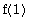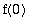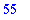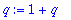Error: too many levels of recursion

It often happens in Maple that one procedure will call a second procedure, which in turn calls a third, and so on. Each time a procedure calls another, some information must be stored in an area of memory called the stack. This is removed when the called procedure returns. The stack has only a finite capacity, and when it is full this error message occurs. The size of the stack may vary depending on the platform, available memory in the computer, and whether or not you are using a student edition of Maple.

In many cases when the error message occurs, it is the result of an infinite recursion. For example:

> f:= n -> f(n-1) + n:

> f(2);

Error, (in f) too many levels of recursion

What happens here is that to evaluate f(2) we need, and to evaluate that we need, and so on forever. No matter how much stack space we have, it will all fill up and result in an error message. A cure for this problem, if we will only want to evaluate the function on positive integers, is to assign f(0) . Then the chain of evaluations, starting at f(n) for any positive integer n , will stop at.

> f(0):= 0:

> f(10);However, if we try f(n) for a sufficiently large n we can still fill up the stack.

> f(1000);

Error, (in f) too many levels of recursion

Another way this error message occurs is as a result of a circular definition.

> q:= 1 + q;

Warning, recursive definition of nameIf the variable q had a value assigned to it, this statement would be perfectly fine: it would simply increase that value by 1. But in this case q did not have a value, and we have a circular definition: in effect we are attempting to define q in terms of itself. The construction itself produces a warning rather than an error, but if you try to evaluate q when it is defined this way the result will be an error.

> q;

Error, too many levels of recursion

When Maple tries to evaluate q , it obtains an expression which itself contains q , then tries to evaluate that, and the process repeats until the stack is filled up.